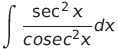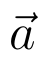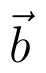Select Page

# 12 Science Maths CBSE Integrals Solutions for MCQ in English

12 Science Maths CBSE Integrals Solutions for MCQ in English to enable students to get Solutions in a narrative video format for the specific question.

Expert Teacher provides 12 Science Maths CBSE Integrals Solutions for MCQ through Video Solutions in English language. This video solution will be useful for students to understand how to write an answer in exam in order to score more marks. This teacher uses a narrative style for a question from Integrals not only to explain the proper method of answering question, but deriving right answer too.

Please find the question below and view the Solution in a narrative video format.

Question:

Solution Video in English:

## Similar Questions from CBSE, 12th Science, Maths, Integrals

Question 1 : Find the integral of the function. (View Answer Video)

Question 2 : Find :(View Answer Video)

Question 3 :(View Answer Video)

Question 4 : Evaluate :(View Answer Video)

Question 5 : Write the value of:(View Answer Video)

### Vector Algebra

Question 1 : Find the area of the triangle with vertices A(1, 1, 2), B(2, 3, 5) and C(1, 5, 5). (View Answer Video)

Question 2 :  Ifanddenote the position vectors of points A and B respectively and C is a point on AB such that AC = 2 CB, then write the position vector of C.  (View Answer Video)

Question 3 : Find the vector quantities :
(i)     Work                     (ii)      Force

Question 4 : Find |a| and |b|, if (a + b).(a - b) = 8 and |a| = 8|b|. (View Answer Video)

Question 5 :  P and Q are two points with position vectorsandrespectively. Write the position vector of a point R which divides the line segment PQ externally in the ratio 2:1.  (View Answer Video)

### Relations and Functions

Question 1 : A functiondefined asis, (View Answer Video)

Question 2 : Consider a binary operation * on N defined as. Choose the correct answer. (View Answer Video)

Question 3 : Let A = {1, 2, 3, 4} and let R = {(2, 2), (3, 3), (4, 4), (1, 2)} be a relation on A. Then, R is, (View Answer Video)

Question 4 : Let * be the binary operation on N given by a * b = LCM of a and b. Find 5 * 7. (View Answer Video)

Question 5 : Let * be the binary operation on N given by a * b = LCM of a and b. Find 20 * 16. (View Answer Video)

### Determinant

Question 1 : Find the value of k, if the area of the triangle is 4 sq unit and vertices are (k, 0) (4, 0) (0, 2). (View Answer Video)

Question 2 : Evaluate. (View Answer Video)

Question 3 : Find the inverse of the matrix. (View Answer Video)

Question 4 : Evaluate the determinant:. (View Answer Video)

Question 5 : Evaluate. (View Answer Video)